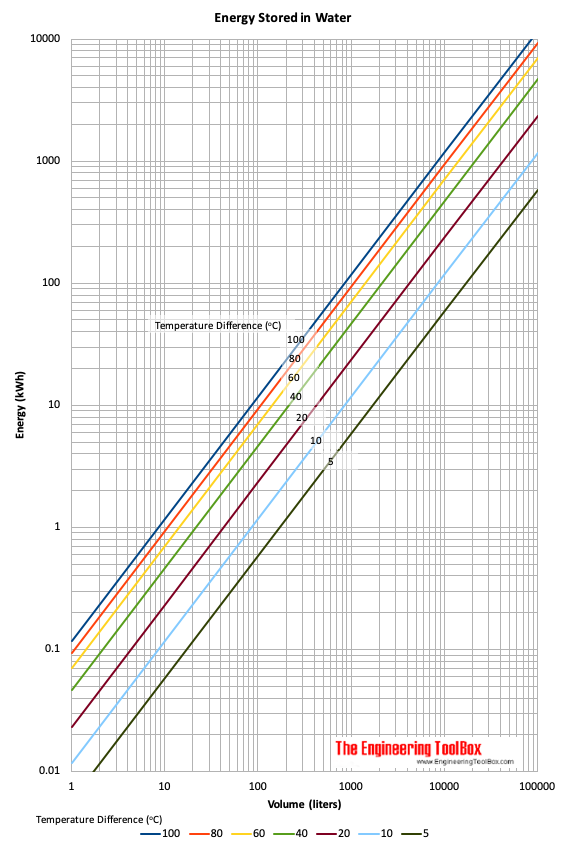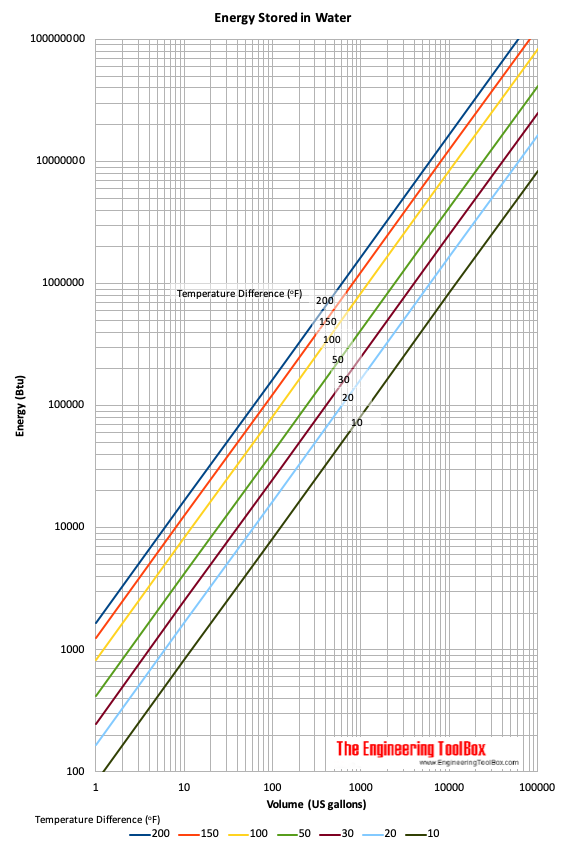Engineering ToolBox - Resources, Tools and Basic Information for Engineering and Design of Technical Applications!

# Energy Accumulated in Heated Water - kWh

## The amount of thermal energy stored in heated water.

Water is often used to store thermal energy. Energy stored - or available - in hot water can be calculated

E = cp dt m                           (1)

where

E = energy (kJ, Btu)

cp = specific heat of water (kJ/kgoC, Btu/lb oF) (4.2 kJ/kgoC, 1 Btu/lbmoF for water)

dt = temperature difference between the hot water and the surroundings (oC, oF))

m = mass of water (kg, lbm)

### Example - Energy stored in a 1000 liter water tank

Water is heated to 90oC. The surrounding temperature (where the energy can be transferred to) is 20oC.

The energy stored in the water tank can be calculated as

E = (4.2 kJ/kgoC) ((90 oC) - (20 oC)) (1000 liter) (1 kg/liter)

= 294000 kJ

= (294000 kWs) (1/3600 h/s)

= 81.7 kWh

• 1 J (Joule) = 0,1020 kpm = 2.778x10-7 kWh = 2.389x10-4 kcal = 0.7376 ft lbf = 1 (kg m2)/s2 = 1 watt second = 1 Nm = 1 ft lb = 9.478x10-4 Btu
• 1 Btu (British thermal unit) = 1055.06 J = 107.6 kpm = 2.92875x10-4 kWh = 251.996 calorie (IT - International Table calorie) = 0.252 kcal = 777.649

### Example - Solar Energy stored in a 200 US gallons Water Tank

A solar energy water buffer tank with 200 US gallons is heated 200oF.

The solar energy stored can be calculated as

E = (1 Btu/lbmoF) (200 oF) (200 US gallons) (8.3 lbm/US gallon)

= 332000 Btu

### Hot Water Energy Calculator

Temperature Difference (oC

Volume (liter)

### Energy Stored in Water - Liters/kWh### Energy Stored in Water - US gallons/Btu's## Engineering ToolBox - SketchUp Extension - Online 3D modeling!

Add standard and customized parametric components - like flange beams, lumbers, piping, stairs and more - to your Sketchup model with the Engineering ToolBox - SketchUp Extension - enabled for use with the amazing, fun and free SketchUp Make and SketchUp Pro .Add the Engineering ToolBox extension to your SketchUp from the SketchUp Pro Sketchup Extension Warehouse!

Translate

## Privacy

We don't collect information from our users. Only emails and answers are saved in our archive. Cookies are only used in the browser to improve user experience.

Some of our calculators and applications let you save application data to your local computer. These applications will - due to browser restrictions - send data between your browser and our server. We don't save this data.

## Citation

• Engineering ToolBox, (2009). Energy Accumulated in Heated Water - kWh. [online] Available at: https://www.engineeringtoolbox.com/energy-storage-water-d_1463.html [Accessed Day Mo. Year].

Modify access date.

. .

#### Scientific Online Calculator6 24